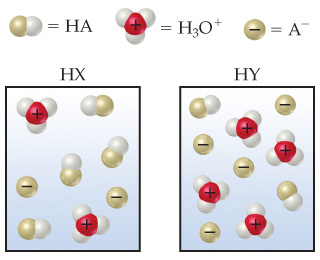⚠️Our tutors found the solution shown to be helpful for the problem you're searching for. We don't have the exact solution yet.

# Solution: The following diagrams represent aqueous solutions of two monoprotic acids, HA (A = X or Y). The water molecules have been omitted for clarity.Which is the stronger acid, HX or HY?

###### Problem
The following diagrams represent aqueous solutions of two monoprotic acids, HA (A = X or Y). The water molecules have been omitted for clarity.Which is the stronger acid, HX or HY?